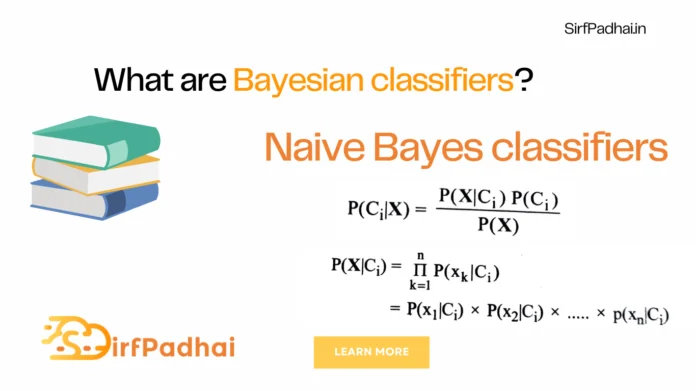# Bayesian classifiers |Naive Bayes classifiers## What are Bayesian classifiers?

Bayesian classifiers are statistical classifiers. They can predict class membership probabilities, such as the probability that a given tuple belongs to a particular class.

Bayesian classification is based on Baye’s theorem. Studies comparing classification algorithms have found a simple Bayesian classifier known as the naive Bayesian classifier to be comparable in performance with decision trees and selected neural network classifiers. Bayesian classifiers have also exhibited high accuracy and speed when applied to large databases.

Naive Bayesian classifiers assume that the effect of an attribute value on a given class is independent of the values of the other attributes. This assumption is called class conditional independence. It is made to simplify the computations involved and, in this sense, is considered ‘naive’. Bayesian belief networks are graphical models, which, unlike naive Bayesian classifiers, allow the representation of dependencies among subsets of attributes. Bayesian belief networks can also be used for classification.

### Explain Naive Bays Classifier with an example.

The Naive Bayesian Classifier or simple Bayesian classifier, works as follows-

(i) Let D be a training set of tuples and their associated class labels. As usual, each tuple is represented by an n-dimensional attribute vector, X = (X1, X2, Xn), depicting n measurements made on the tuple from n attributes, respectively, A1, A2, …., An.

(ii) Suppose that there are m classes, C1, C2, ….., Cm. Given a tuple, X, the classifier will predict that X belongs to the class having the highest posterior probability, conditioned on X. That is, the naive Bayesian classifier predicts that tuple X belongs to the class Ci if the only if

P(Ci|X)>P(Cj|X)  for  1 ≤ j ≤ m, j≠i

Thus we maximize P(Ci|X|). The class Ci for which P(Ci|X) is maximized is called the maximum posteriori hypothesis. By Baye’s theorem

(iii) As P(X) is constant for all classes, only P(X|Ci) P(Ci) need be maximized. If the class prior probabilities are not known, then it is commonly assumed that the classes are equally likely, that is, P(C1) = P(C2) = …… = P(Cm), and we would therefore maximize P(X|Ci). Otherwise, we maximize P(X|Ci) P(Ci).

(iv) Given data sets with many attributes, it would be extremely computationally expensive to compute P(X|Ci). In order to reduce computation in evaluating P(X|Ci), the naive assumption of class conditional independence is made. This presumes that the values of the attributes are conditionally independent of one another, given the class label of the tuple. Thus,

(v) In order to predict the class label of X, P(X|Ci) P(Ci) is evaluated for each class C¡. The classifier predicts that the class label of tuple X is the class Ci if and only if

P(X|Ci) P(Ci) > P(X|Cj) P(Cj) for 1 ≤ j ≤ m, j ≠ i

In other words, the predicted class label is the class Ci for which P(X|Ci) P(Ci) is the maximum.

Previous articleExplain the term training test and validation sets.
Next articleProbability Density Function